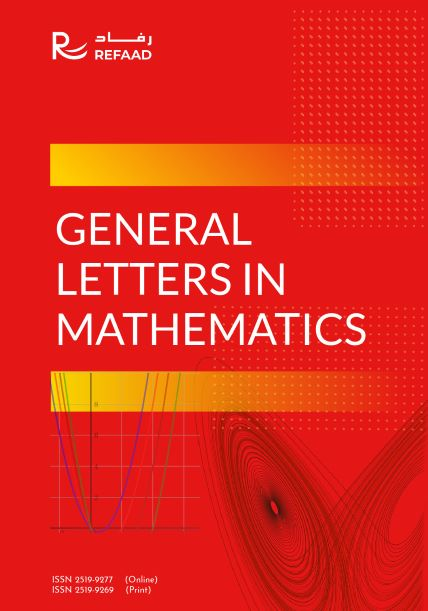General Letters in Mathematics (GLM) is a peer-reviewed, open access journal which provides an original research papers in all aspects of Mathematics, and is published Quarterly by Refaad. In particular, (GLM) Journal intends to offer mathematicians from middle east and all arab countries a particular opportunity to circulate the results of their researches though it is open to contribution from mathematicians across the world.

#### 30 (Days)

Median time to first decision
Latest Articles

# Epsilon-Diskcyclic Operators

In this paper, we will introduce a new concept of cyclic phenomena that is called "-diskcyclic operator and construct examples to show the relationship between other types. In particular, for each " in (0, 1), we will construct an "-diskcyclic operator that is not diskcyclic. These examples illustrate ...

# New search direction of steepest descent method for solving large linear systems

The steepest descent (SD) method is well-known as the simplest method in optimization. In this paper, we propose a new SD search direction for solving system of linear equations Ax = b. We also prove that the proposed SD method with exact line search satisfies descent condition and possesses global ...

# A Stochastic Maximum Principle for a Minimization Problem Under Partial Information

Eric.K Tatiagoum

In this paper, we establish a stochastic maximum principle for a stochastic minimization problem under partial information. With the Backward stochastic differential equations (in short BSDE&rsquo;s), we establish a sufficient condition of optimality to characterize and determine an optimal control. ...

# Soft Pre Separation Axioms and Function with Soft Pre Closed Graph

Nehmat K. Ahmed , Alias B. Khalaf

Several notions on soft topology are studied and their basic properties are investigated by using the concept of soft pre open sets and soft pre closure operator which are derived from the basics of soft set theory established by Molodtsov . In this paper we introduce some soft separation axioms ...

# Existence and Ulam stability of solutions for Caputo-Hadamard fractional differential equations

In this paper, we study the existence of solutions for fractional differential equations with the Caputo-Hadamard fractional derivative of order 2 (1, 2]. The uniqueness result is proved via Banach&rsquo;s contraction mapping principle and the existence results are established by using the Schauder&rsquo;s ...